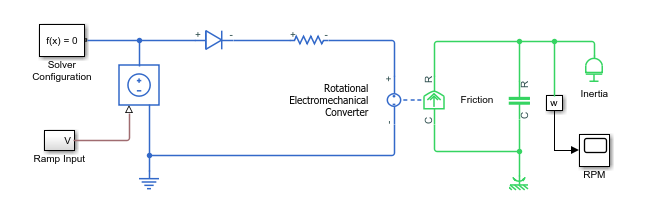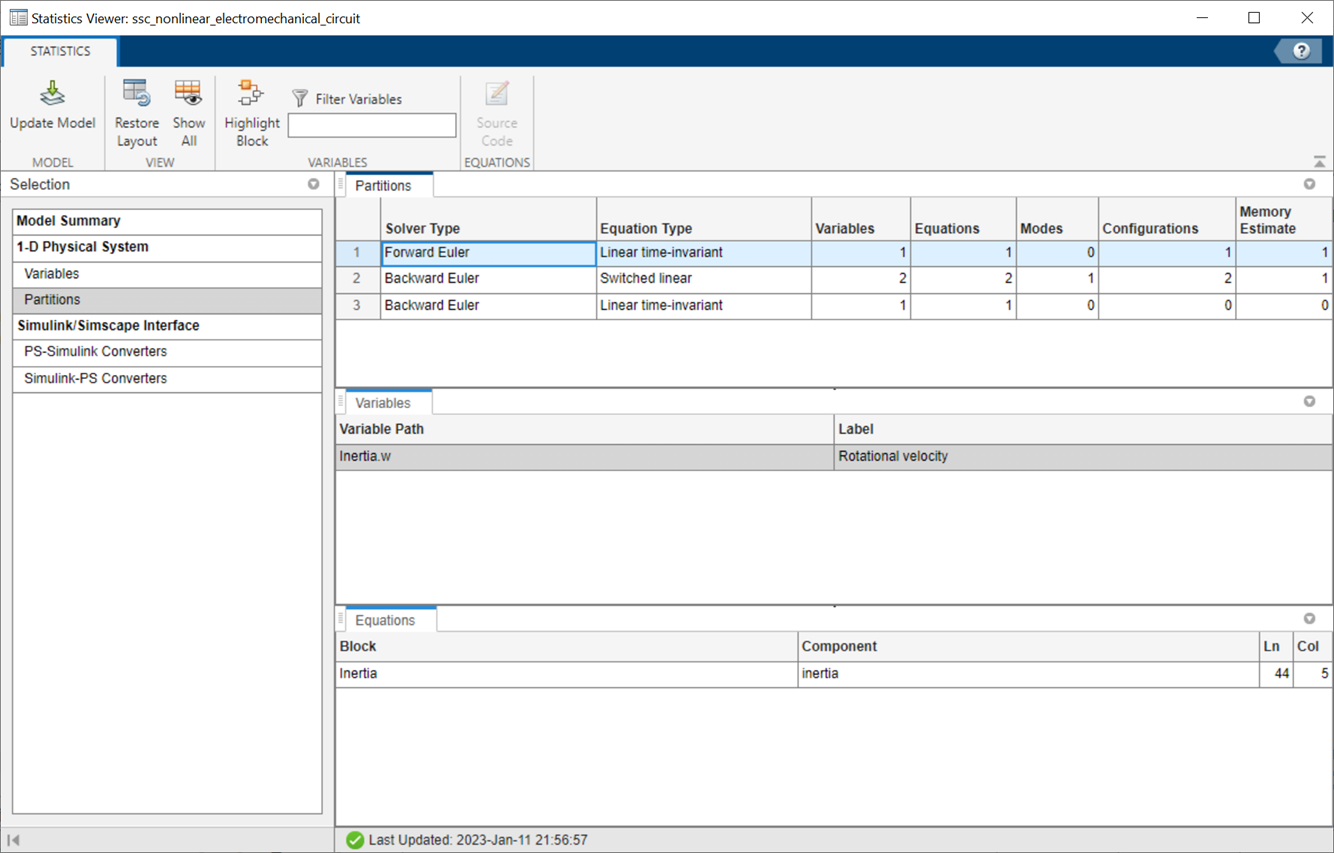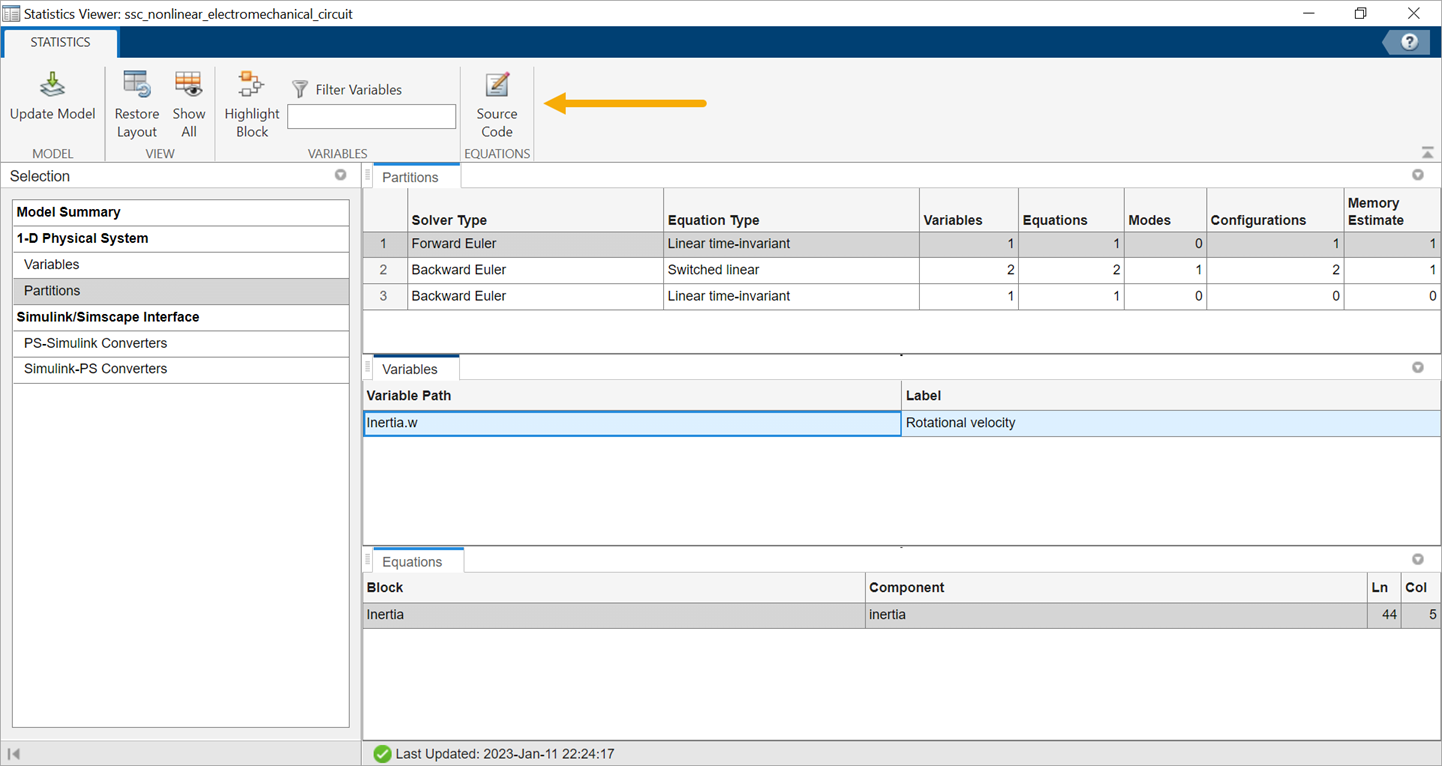## Understanding How the Partitioning Solver Works

This topic uses the Nonlinear Electromechanical Circuit with Partitioning Solver example to provide an in-depth look into the Partitioning solver functionality. It examines the different types of partitions and their equations and explains how the Partitioning solver solves them to yield faster simulation.

1. To open the Nonlinear Electromechanical Circuit with Partitioning Solver example model, type `ssc_nonlinear_electromechanical_circuit` in the MATLAB® Command Window.2. To view model statistics, in the model window, on the Debug tab, click Simscape > Statistics Viewer. Click the button in the toolbar of the viewer window, if necessary, to populate the viewer with data.

3. Expand the Number of partitions node.It shows that the solver divides the system into three partitions. The first partition is solved using the Forward Euler method, and the other two partitions are solved using the Backward Euler method.

4. By selecting Number of variables under each of the partition nodes, you can see the names of the variables that belong to that partition.Partition 1 owns the `Inertia.w` variable, which represents the rotational velocity of the Inertia block.

Partition 2 owns `Diode.v` and ```Sensing.Ideal Rotational Motion Sensor.phi```, and Partition 3 owns `Inertia.t`. Each partition is responsible for updating the values of the state variables it owns.

5. The Statistics Viewer also contains links to equations in each partition.For example, if you select Number of equations under Partition 1, and then click the `ssc_nonlinear_electromechanical_circuit/Inertia` link under Source, the source code for the Inertia blocks opens in the MATLAB editor, pointing to this equation:

```t == inertia * w.der; ```

Similarly, you can see the equations for other partitions.

The Partitioning solver assembles all these equations into the system of equations required to simulate the model:Here, Sensor.phi is the abbreviation of the ```Sensing.Ideal Rotational Motion Sensor.phi``` variable (used to make the presentation of the system of equations more compact). `m0` is the boolean originating from the equation in the Diode block, where `Diode.v` is compared with the Forward voltage:

``` if v > Vf i == (v - Vf*(1-Ron*Goff))/Ron; else i == v*Goff; end ```

Comparing this system of equations with the Statistics Viewer data, you can see that the first row of the system is in Partition 3, because Partition 3 owns the `Inertia.t` state variable. Similarly, the second and third rows are in Partition 2, and the fourth row is in Partition 1.

The equation type of a partition depends only on the terms involving owned states and is not affected by the connection function. For example, Partition 3 lists its Equation Type as Linear time-invariant, despite having nonlinearities in the connection function term, because it is linear time-invariant with respect to its owned states.

During simulation, the Partitioning solver solves the partitions in the same order in which they are listed in the Statistics Viewer (that is, bottom-up in the system of equations), using the specified method (Forward Euler or Backward Euler). The solver uses the updated state values, obtained after solving each partition, to perform state update for upstream partitions.